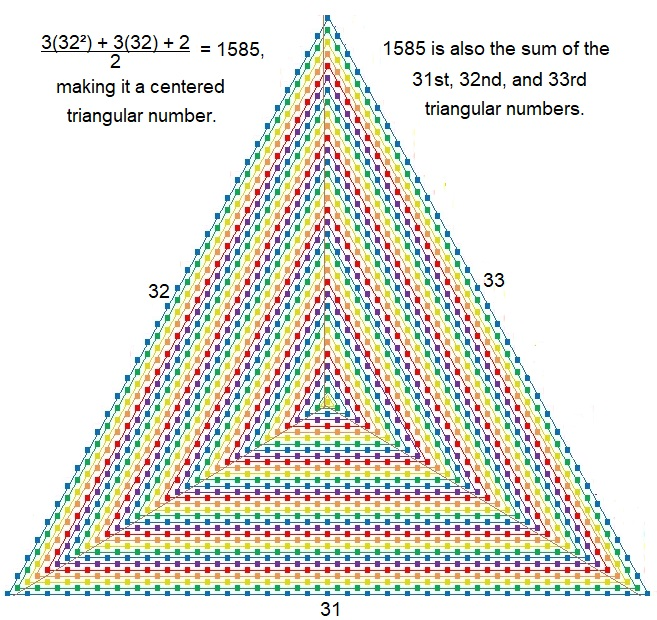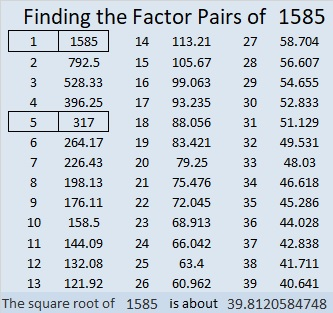# 1585 is a Centered Triangular Number

Contents

### Today’s Puzzle:

What geometric shape can 1585 tiny squares be arranged into?

If you answered a 5 × 317 rectangle, you would be right, but there is another shape that is probably more interesting than that rectangle: 1585 tiny squares can be arranged into a centered triangle as illustrated below.Can you see the three consecutive triangular numbers that make up this centered triangle?

### Factors of 1585:

• 1585 is a composite number.
• Prime factorization: 1585 = 5 × 317.
• 1585 has no exponents greater than 1 in its prime factorization, so √1585 cannot be simplified.
• The exponents in the prime factorization are 1 and 1. Adding one to each exponent and multiplying we get (1 + 1)(1 + 1) = 2 × 2 = 4. Therefore 1585 has exactly 4 factors.
• The factors of 1585 are outlined with their factor pair partners in the graphic below.### More about the Number 1585:

1585 is the sum of two squares in two different ways:
39² + 8² = 1585
36² + 17² = 1585

1585 is the hypotenuse of FOUR Pythagorean triples:
375-1540-1585, which is 5 times (75-308-317),
624-1457-1585, calculated from 2(39)(8), 39² – 8², 39² + 8²
951-1268-1585, which is (3-4-5) times 317, and
1007-1224-1585, calculated from 36² – 17², 2(36)(17), 36² + 17².

## 3 thoughts on “1585 is a Centered Triangular Number”

1.ShiraDest

Very cool! 🙂

•ivasallay

Thank you!

•ShiraDest

You’re welcome!

This site uses Akismet to reduce spam. Learn how your comment data is processed.